# GSEB Solutions Class 7 Maths Chapter 2 Fractions and Decimals Ex 2.2

Gujarat Board GSEB Textbook Solutions Class 7 Maths Chapter 2 Fractions and Decimals Ex 2.2 Textbook Questions and Answers.

## Gujarat Board Textbook Solutions Class 7 Maths Chapter 2 Fractions and Decimals Ex 2.2Question 1.
Which of the drawings (a) to (d) show:
(i) 2 x $$\frac { 1 }{ 5 }$$
(ii) 2 x $$\frac { 1 }{ 2 }$$
(iii) 3 x $$\frac { 2 }{ 3 }$$
(iv) 3 x $$\frac { 1 }{ 4 }$$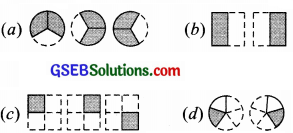Solution:
(i) 2 x $$\frac { 1 }{ 5 }$$
= $$\frac { 1 }{ 5 }$$ + $$\frac { 1 }{ 5 }$$, which is represented by the drawing (d).
Thus (i) → (d).

(ii) 2 x $$\frac { 1 }{ 2 }$$
= $$\frac { 1 }{ 2 }$$ + $$\frac { 1 }{ 2 }$$, which is represented by the drawing (d).
Thus (ii) → (d).

(iii) 3 x $$\frac { 2 }{ 3 }$$
$$\frac { 2 }{ 3 }$$ + $$\frac { 2 }{ 3 }$$ + $$\frac { 2 }{ 3 }$$, which is represented by the drawing (a).
Thus, (iii) → (a).

(iv) 3 x $$\frac { 1 }{ 4 }$$
$$\frac { 1 }{ 4 }$$ + $$\frac { 1 }{ 4 }$$ + $$\frac { 1 }{ 4 }$$, which is represented by the drawing (c).
Thus, (iv) → (c).Question 2.
Some pictures (a) to (c) are given below. Tell which of them show:
(i) 3 x $$\frac { 1 }{ 5 }$$
(ii) 2 x $$\frac { 1 }{ 3 }$$ = $$\frac { 2 }{ 3 }$$
(iii) 2 x $$\frac { 1 }{ 3 }$$ = 2 x $$\frac { 1 }{ 4 }$$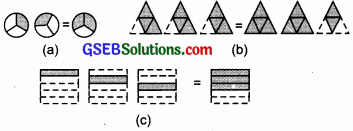Solution:
(i) ∵ 3 x = $$\frac { 1 }{ 5 }$$
$$\frac { 1 }{ 5 }$$ + $$\frac { 1 }{ 5 }$$ + $$\frac { 1 }{ 5 }$$ = $$\frac { 3 }{ 5 }$$, which is shown by the drawing (c).
∴ (i) → (C)

(ii) ∵ 2 x $$\frac { 1 }{ 3 }$$ = $$\frac { 1 }{ 3 }$$ + $$\frac { 1 }{ 3 }$$ = $$\frac { 2 }{ 3 }$$, which is shown by the drawing (a).
∴ (ii) → (C)

(iii) ∵ 3 x $$\frac { 3 }{ 4 }$$ = $$\frac { 3 }{ 4 }$$ + $$\frac { 3 }{ 4 }$$ + $$\frac { 3 }{ 4 }$$, which is shown by the drawing (a).
∴ (iii) → (C)

Question 3.
Multiply and reduce to lowest form and convert into a mixed fraction:
(i) 7 x $$\frac { 3 }{ 5 }$$
(ii) 4 x $$\frac { 1 }{ 3 }$$
(iii) 2 x $$\frac { 6 }{ 7 }$$
(iv) 5 x $$\frac { 2 }{ 9 }$$
(v) $$\frac { 2 }{ 3 }$$ x 4
(vi) $$\frac { 5 }{ 2 }$$ x 6
(vii) 11 x $$\frac { 4 }{ 7 }$$
(viii) 20 x $$\frac { 4 }{ 5 }$$
(ix) 13 x $$\frac { 1 }{ 3 }$$
(x) 15 x $$\frac { 3 }{ 5 }$$
Solution: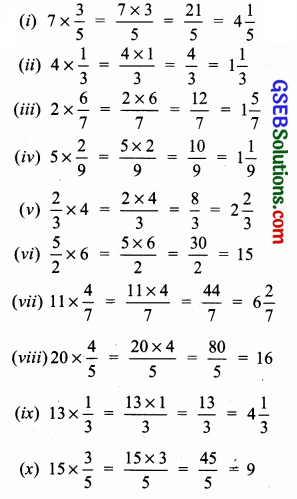Question 4.
(i) $$\frac { 1 }{ 2 }$$ of the circles in box (a)
(ii) $$\frac { 2 }{ 3 }$$ of the triangles in box (b)
(ii) $$\frac { 3 }{ 5 }$$ of the squares in box (c)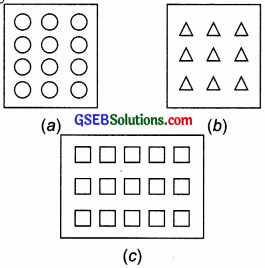Solution:
(i) $$\frac { 1 }{ 2 }$$ of the circles:
∵ $$\frac { 1 }{ 2 }$$ of 12 = $$\frac { 1 }{ 2 }$$ x 12 = 6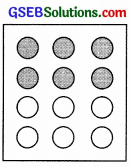(ii) $$\frac { 2 }{ 3 }$$ of the triangles:
∵ $$\frac { 2 }{ 3 }$$ of 9 = $$\frac { 2 × 9 }{ 3 }$$ = 6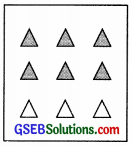(ii) $$\frac { 3 }{ 5 }$$ of the squares:
∵ $$\frac { 3 }{ 5 }$$ or 15 = $$\frac { 3 × 15 }{ 5 }$$ = 9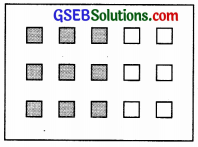Question 5.
Find:
(a) $$\frac { 1 }{ 2 }$$ of (i) 24 (ii) 46
(b) $$\frac { 2 }{ 3 }$$ of (i) 18 (ii) 27
(c) $$\frac { 3 }{ 4 }$$ of (i) 16 (ii) 36
(d) $$\frac { 4 }{ 5 }$$ of (i) 20 (ii) 35
Solution: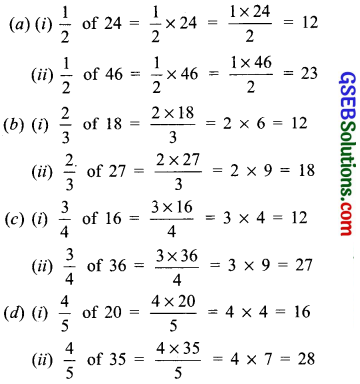Question 6.
Multiply and express as a mixed fraction:
(a) 3 x 5$$\frac { 1 }{ 5 }$$
(b) 5 x 6$$\frac { 3 }{ 4 }$$
(c) 7 x 2$$\frac { 1 }{ 4 }$$
(d) 4 x 6$$\frac { 1 }{ 3 }$$
(e) 3$$\frac { 1 }{ 4 }$$ x 6
(f) 3$$\frac { 2 }{ 5 }$$ x 8
Solution: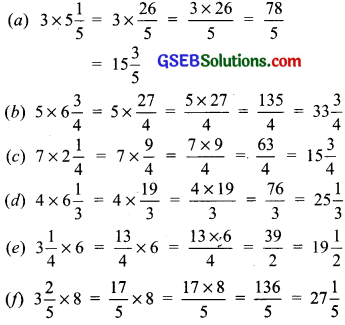Question 7.
Find:
(a) $$\frac { 1 }{ 2 }$$ of (i) 2$$\frac { 3 }{ 4 }$$ (ii) 4$$\frac { 2 }{ 9 }$$
(b) $$\frac { 5 }{ 8 }$$ of (i) 3$$\frac { 5 }{ 6 }$$ (ii) 9$$\frac { 2 }{ 3 }$$
Solution:
(a) (i) $$\frac { 1 }{ 2 }$$ of 2$$\frac { 3 }{ 4 }$$
= $$\frac { 1 }{ 2 }$$ of $$\frac { 11 }{ 4 }$$
= $$\frac { 1×11 }{ 2×4 }$$
= $$\frac { 11 }{ 8 }$$
= 1$$\frac { 3 }{ 8 }$$

(ii) $$\frac { 1 }{ 2 }$$ of 4$$\frac { 2 }{ 9 }$$
= $$\frac { 1 }{ 2 }$$ of $$\frac { 38 }{ 9 }$$
= $$\frac { 1×38 }{ 2×9 }$$
= $$\frac { 19 }{ 9 }$$
= 2$$\frac { 1 }{ 9 }$$

(b) (i) $$\frac { 5 }{ 8 }$$ of 3$$\frac { 5 }{ 6 }$$
= $$\frac { 5 }{ 8 }$$ of $$\frac { 23 }{ 6 }$$
= $$\frac { 5×23 }{ 8×6 }$$
= $$\frac { 115 }{ 48 }$$
= 2$$\frac { 19 }{ 48 }$$

(ii) $$\frac { 5 }{ 8 }$$ of 9$$\frac { 2 }{ 3 }$$
= $$\frac { 5 }{ 8 }$$ of $$\frac { 29 }{ 3 }$$
= $$\frac { 5×29 }{ 8×3 }$$
= $$\frac { 145 }{ 24 }$$
= 6$$\frac { 1 }{ 24 }$$Question 8.
Vidya and Pratap went for a picnic. Their mother gave them a water bottle that contained 5 litres of water. Vidya consumed $$\frac { 2 }{ 5 }$$ of the water.
Pratap consumed the remaining water.
(i) How much water did Vidya drink7
(ii) What fraction of the total quantity of water did Pratap drink?
Solution:
Total quantity of water = 5 litres
(i) Amount of water consumed by Vidya = $$\frac { 2 }{ 5 }$$ of 5 litres = $$\frac { 2 }{ 5 }$$ x 5 litres = 2 litres
(ii) Remaining water = water consumed by Pratap
i.e. Amount of water consumed by Pratap = 5 litres – 2 litres = 3 litres
Fraction of water consumed by Pratap = $$\frac { 3 }{ 5 }$$